US20040004748A1 - Optical scanner - Google Patents

Info

Publication number
US20040004748A1
US20040004748A1 US10/461,488 US46148803A US2004004748A1 US 20040004748 A1 US20040004748 A1 US 20040004748A1 US 46148803 A US46148803 A US 46148803A US 2004004748 A1 US2004004748 A1 US 2004004748A1
Authority
US
United States
Prior art keywords
lens
light beam
nm
represents
optical system
Prior art date
Legal status (The legal status is an assumption and is not a legal conclusion. Google has not performed a legal analysis and makes no representation as to the accuracy of the status listed.)
Granted
Application number
US10/461,488
Other versions
US6771429B2 (en
Inventor
Motonobu Kawabata
Masahiko Kokubo
Current Assignee (The listed assignees may be inaccurate. Google has not performed a legal analysis and makes no representation or warranty as to the accuracy of the list.)
Screen Holdings Co Ltd
Original Assignee
Screen Holdings Co Ltd
Priority date (The priority date is an assumption and is not a legal conclusion. Google has not performed a legal analysis and makes no representation as to the accuracy of the date listed.)
Filing date
Publication date
Priority to JPP2002-186120 priority Critical
Priority to JP2002186120A priority patent/JP2004029416A/en
Application filed by Screen Holdings Co Ltd filed Critical Screen Holdings Co Ltd
Assigned to DAINIPPON SCREEN MFG CO., LTD. reassignment DAINIPPON SCREEN MFG CO., LTD. ASSIGNMENT OF ASSIGNORS INTEREST (SEE DOCUMENT FOR DETAILS). Assignors: KAWABATA, MOTONOBU, KOKUBO, MASAHIKO
Publication of US20040004748A1 publication Critical patent/US20040004748A1/en
Publication of US6771429B2 publication Critical patent/US6771429B2/en
Application granted granted Critical
Assigned to SCREEN Holdings Co., Ltd. reassignment SCREEN Holdings Co., Ltd. CHANGE OF NAME (SEE DOCUMENT FOR DETAILS). Assignors: DAINIPPON SCREEN MFG. CO., LTD.
Application status is Expired - Fee Related legal-status Critical
Anticipated expiration legal-status Critical

Images

•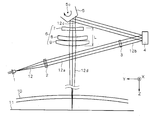•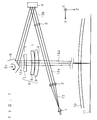•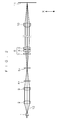•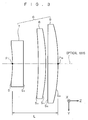•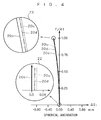••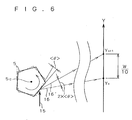•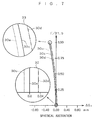•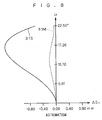•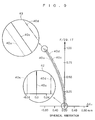•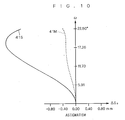•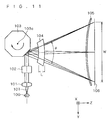•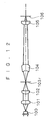•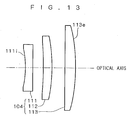Classifications

• GPHYSICS
• G02OPTICS
• G02BOPTICAL ELEMENTS, SYSTEMS, OR APPARATUS
• G02B27/00Other optical systems; Other optical apparatus
• G02B27/0025Other optical systems; Other optical apparatus for optical correction, e.g. distorsion, aberration
• G02B27/0031Other optical systems; Other optical apparatus for optical correction, e.g. distorsion, aberration for scanning purposes
• GPHYSICS
• G02OPTICS
• G02BOPTICAL ELEMENTS, SYSTEMS, OR APPARATUS
• G02B26/00Optical devices or arrangements using movable or deformable optical elements for controlling the intensity, colour, phase, polarisation or direction of light, e.g. switching, gating, modulating
• G02B26/08Optical devices or arrangements using movable or deformable optical elements for controlling the intensity, colour, phase, polarisation or direction of light, e.g. switching, gating, modulating for controlling the direction of light
• G02B26/10Scanning systems
• G02B26/12Scanning systems using multifaceted mirrors
• G02B26/124Details of the optical system between the light source and the polygonal mirror

Abstract

An optical scanner according to the present invention comprises a first imaging optical system consisting of a collimator lens and a cylindrical lens, a light deflector reflecting/deflecting a light beam for scanning, and a second imaging optical system consisting of an f-θ lens and an anamorphic lens. The f-θ lens has three groups of lenses, i.e., a first lens having negative refracting power, a second lens having positive refracting power and a third lens having positive refracting power. The f-θ lens is formed to satisfy relational expressions L/f<0.100 and 0.10≧r1/r3≦0.26, where L represents the total length of the f-θ lens, f represents the focal distance of the f-θ lens, r1 represents the radius of curvature of a light beam entrance-side refracting interface of the first lens and r3 represents the radius of curvature of a light beam entrance-side refracting interface of the second lens. Thus, a compact optical scanner comprising an f-θ lens having a small total length L and high optical performance is provided also when increasing a focal length f as well as a scanning line length W.

Description

BACKGROUND OF THE INVENTION
• 1. Field of the Invention 
• The present invention relates to an optical scanner reflecting/deflecting a light beam such as a laser beam for scanning an object. 
• 2. Description of the Background Art 
• In general, a two-dimensional image apparatus such as a laser printer or a scanner is mounted with an optical scanner precisely scanning an object with a laser beam. 
• This type of optical scanner reflects/deflects the laser beam with a light deflector such as a galvanometer mirror or a polygon mirror for scanning an objective surface of a photosensitive drum or the like. While the light deflector rotates at an equiangular velocity, the laser beam must scan the objective surface at a uniform rate. Therefore, the optical scanner employs an f-θ (ef-theta) lens as an optical system letting the laser beam reflected/deflected by the light deflector scan the objective surface at a uniform rate. The f-θ lens is an optical system having a distortion characteristic satisfying y=fω) (f: focal distance, ω: half angle of view) in relation to an ideal image height y. 
• FIGS. 11 and 12 show a conventional optical scanner mounted with an f-θ lens  104. FIG. 11 is a schematic block diagram of the optical scanner developed along a Y-Z plane, and FIG. 12 is a longitudinal sectional view developing the optical scanner shown in FIG. 11 along an optical axis. Referring to FIGS. 11 and 12, numeral 100 denotes a light source (semiconductor laser), numeral 101 denotes a collimator lens, numeral 102 denotes a cylindrical lens, numeral 103 denotes a polygon mirror, numeral 104 denotes the f-θ lens, numeral 105 denotes an anamorphic lens and numeral 106 denotes an objective surface. Directions X, Y and Z shown in FIGS. 11 and 12 are perpendicular to each other.
• The light source  100 oscillates a laser beam 107 directly modulated by a driving circuit (not shown). This laser beam 107 is parallelized by the collimator lens 101 and converged by the cylindrical lens 102 for forming a linear image on a reflecting surface 103 r of the polygon mirror 103. The polygon mirror 103 rotates about a rotational axis 103 c by tens of thousands of revolutions per minute and the f-θ lens 104 is an optical system converting equiangular velocity motion of incident light from the reflecting surface 103 r to uniform motion, whereby a light beam reflected by the reflecting surface 103 r of the polygon mirror 103 is deflected at an equilateral velocity and scans the objective surface 106 in the direction Y. The anamorphic lens 105 converges light incident from the f-θ lens 104 perpendicularly (direction X) to a primary scanning direction (direction Y) for forming an image on the objective surface 106. As shown in FIG. 11, the light beam scans the objective surface 106 over a scanning line length W, and hence the f-θ lens 104 must have a wide total angle θ of view. Further, the size of an image has recently been so increased that an optical scanner having a large scanning line length W is required. Assuming that f represents the focal distance of the f-θ lens 104 at the working wavelength for the light beam, the following relational expression holds:
• W=fθ
• When the scanning line length W is enlarged while keeping the total angle θ of view constant, therefore, the focal distance f of the f-θ lens  104 is increased. In order to enlarge the scanning line length W while keeping the focal distance f of the f-θ lens 104 constant, on the other hand, the total angle θ of view must be increased. In this case, the aperture of the f-θ lens 104 is so increased that it is difficult to precisely work the f-θ lens 104 and correct optical aberration values thereof, to readily increase the cost for the f-θ lens 104.
• Compactification of the optical scanner has also been required in recent years. As shown in FIG. 13, an f-θ lens  104 built in the optical scanner is formed by three groups of lenses, i.e., a first lens 111 having negative refracting power, a second lens 112 having positive refracting power an a third lens 113 having positive refracting power. Between the total length L (face-to-face distance between an entrance-side curved surface 1 i of the first lens 111 and an exit-side curved surface 113 e of the third lens 113) of the f-θ) lens 104 and a focal distance f, the following relational expression holds:
• 0.100<L/f<0.108
• Hence, the total length L exceeds 0.100×f. An f-θ) lens having optical performance not deteriorated also when the total length L is further reduced has recently been required. 
• SUMMARY OF THE INVENTION
• The present invention is directed to an optical scanner reflecting/deflecting a light beam such as a laser beam for scanning an object. 
• According to the present invention, the optical scanner comprises a light deflector periodically reflecting a light beam emitted from a light source to periodically deflect said light beam and an imaging optical system having such a distortion characteristic that the product of a focal distance and a half angle of view defines an ideal image height for imaging the light beam deflected by the light deflector on an objective surface, and the imaging optical system comprises a first lens having negative refracting power, a second lens having positive refracting power and a third lens having positive refracting power successively from an entrance side for the light beam to satisfy the following expressions (1) and (2):  $L f < 0.100 ( 1 ) 0.10 ≤ r1 r3 ≤ 0.26 ( 2 )$
• where L represents the length between a plane of incidence of the first lens and a plane of exit of the third lens along an optical axis direction and f represents the composite focal distance of the first lens, the second lens and the third lens in the above expression (1) while r1 represents the radius of curvature of a refracting interface on the entrance side for the light beam in the first lens and r3 represents the radius of curvature of a refracting interface on the entrance side for the light beam in the second lens in the above expression (2). 
• A compact imaging optical system can be formed with a total length L smaller as compared with a focal distance f by satisfying the above expression (1), thereby implementing a compact optical scanner. Further, the imaging optical system can properly correct bending of a meridional image surface by satisfying the above expression (2). According to the present invention, both conditions of the above expressions (1) and (2) are compatible with each other, whereby a compact optical scanner having high optical performance can be manufactured. 
• Preferably, the first lens, the second lens and the third lens are made of an optical material satisfying the following expression (4) on the basis of a partial Abbe's number ν defined in the following expression (3):  $υ = N A - 1 N MIN - N MAX ( 3 ) 1.40 ≤ υ p   s υ n   g ≤ 1.70 ( 4 )$
• where N A represents a refractive index with respect to the central wavelength of a working wave range of the light beam, NMIN represents a refractive index with respect to the lower limit of the working wave range of the light beam and NMAX represents a refractive index with respect to the upper limit of the working wave range of the light beam in the above expression (3) while νps represents the partial Abbe's number of the second lens and the third lens and vng represents the partial Abbe's number of the first lens in the above expression (4).
• An imaging optical system capable of correcting on-axis chromatic aberration within tolerance can be implemented by satisfying the above expression (4). 
• More preferably, the imaging optical system satisfies the following expression (5):  $0.26 ≤  f1  f ≤ 0.33 ( 5 )$
• where f1 represents the focal distance of the first lens in the above expression (5). 
• An imaging optical system capable of further properly correcting bending of a meridional image surface can be implemented by satisfying the above expression (5). 
• More preferably, the imaging optical system is formed to satisfy the following expression (6):  $0.41 ≤ f3 f ≤ 0.66 ( 6 )$
• where f3 represents the focal distance of the third lens in the above expression (6). 
• An imaging optical system capable of improving a scanning property of a light beam can be implemented by satisfying the above expression (6). 
• More preferably, another imaging optical system converging the light beam emitted from the light source only in the direction of the rotational axis of the light deflector and imaging the light beam on a reflecting surface of the light deflector is further provided on an optical path between the light source and the light deflector, and the imaging optical system further comprises an anamorphic lens converging a light beam outgoing from the third lens in the direction of the rotational axis of the light deflector and imaging the light beam on the objective surface. 
• When displacement is present in perpendicularity of the reflecting surface of the light deflector, inclination of reflected light resulting from this displacement can be so corrected that the light beam can precisely scan the objective surface at a regular pitch. 
• When employing a light beam having a central wavelength of around 405 nm, the imaging optical system more preferably satisfies the following expression (2A):  $0.10 ≤ r1 r3 ≤ 0.26 ( 2  A )$
• A compact imaging optical system capable of properly correcting bending of a meridional image surface can be implemented particularly with respect to the light beam having the central wavelength of around 405 nm. 
• When employing the light beam having the central wavelength of around 405 nm, the optical material more preferably satisfies the following expression (4A) with respect to the light beam having the central wavelength of around 405 nm:  $1.44 ≤ υ p   s υ n   g ≤ 1.70 ( 4  A )$
• An imaging optical system capable of correcting on-axis chromatic aberration within a proper range can be implemented particularly with respect to the light beam having the central wavelength of around 405 nm. 
• When employing the light beam having the central wavelength of around 405 nm, the imaging optical system more preferably satisfies the following expression (5A) with respect to the light beam having the central wavelength of around 405 nm:  $0.28 ≤  f1  f ≤ 0.33 ( 5  A )$
• An imaging optical system capable of properly correcting bending of a meridional image surface can be implemented particularly with respect to the light beam having the central wavelength of around 405 nm. 
• When employing a light beam having a central wavelength of around 635 nm, the imaging optical system more preferably satisfies the following expression (2B):  $0.11 ≤ r1 r3 ≤ 0.25 ( 2  B )$
• A compact imaging optical system capable of properly correcting bending of a meridional image surface can be implemented particularly with respect to the light beam having the central wavelength of around 635 nm. 
• When employing the light beam having the central wavelength of around 635 nm, the optical material more preferably satisfies the following expression (4B) with respect to the light beam having the central wavelength of around 635 nm:  $1.50 ≤ υ p   s υ n   g ≤ 1.62 ( 4  B )$
• An imaging optical system capable of correcting on-axis chromatic aberration within a proper range can be implemented particularly with respect to the light beam having the central wavelength of around 635 nm. 
• When employing the light beam having the central wavelength of around 635 nm, the imaging optical system more preferably satisfies the following expression (5B) with respect to the light beam having the central wavelength of around 635 nm:  $0.30 ≤  f1  f ≤ 0.33 ( 5  B )$
• An imaging optical system capable of properly correcting bending of a meridional image surface can be implemented particularly with respect to the light beam having the central wavelength of around 635 nm. 
• When employing a light beam having a central wavelength of around 785 nm, the imaging optical system more preferably satisfies the following expression (2C):  $0.12 ≤ r1 r3 ≤ 0.21 ( 2  C )$
• A compact imaging optical system capable of properly correcting bending of a meridional image surface can be implemented particularly with respect to the light beam having the central wavelength of around 785 nm. 
• When employing the light beam having the central wavelength of around 785 nm, the optical material more preferably satisfies the following expression (4C) with respect to the light beam having the central wavelength of around 785 nm:  $1.40 ≤ υ p   s υ n   g ≤ 1.70 ( 4  C )$
• An imaging optical system capable of correcting on-axis chromatic aberration within a proper range can be implemented particularly with respect to the light beam having the central wavelength of around 785 nm. 
• When employing the light beam having the central wavelength of around 785 nm, the imaging optical system more preferably satisfies the following expression (5C) with respect to the light beam having the central wavelength of around 785 nm:  $0.26 ≤  f1  f ≤ 0.31 ( 5  C )$
• An imaging optical system capable of properly correcting bending of a meridional image surface can be implemented particularly with respect to the light beam having the central wavelength of around 785 nm. 
• Accordingly, an object of the present invention is to provide a compact optical scanner comprising an f-θ lens having a small total length L and high optical performance also when a focal distance f as well as a scanning line length W are increased. 
• The foregoing and other objects, features, aspects and advantages of the present invention will become more apparent from the following detailed description of the present invention when taken in conjunction with the accompanying drawings.
• BRIEF DESCRIPTION OF THE DRAWINGS
• FIG. 1 is a schematic diagram showing the overall structure of an optical scanner according to each embodiment of the present invention; 
• FIG. 2 is a vertical sectional view developing the optical scanner shown in FIG. 1 along an optical axis; 
• FIG. 3 is a schematic sectional view of an f-θ lens according to each embodiment; 
• FIG. 4 is a spherical aberration diagram of an f-θ lens according to a first embodiment of the present invention; 
• FIG. 5 is an astigmatism diagram of the f-θ lens according to the first embodiment; 
• FIG. 6 is a diagram for illustrating f-θ characteristics; 
• FIG. 7 is a spherical aberration diagram of an f-θ lens according to a second embodiment of the present invention; 
• FIG. 8 is an astigmatism diagram of the f-θ lens according to the second embodiment; 
• FIG. 9 is a spherical aberration diagram of an f-θ lens according to a third embodiment of the present invention; 
• FIG. 10 is an astigmatism diagram of the f-θ lens according to the third embodiment; 
• FIG. 11 is a diagram showing a conventional optical scanner developed along a Y-Z plane; 
• FIG. 12 is a longitudinal sectional of the optical scanner shown in FIG. 11 developed along an optical axis; and 
• FIG. 13 is a schematic sectional view showing a conventional f-θ lens.
• DESCRIPTION OF THE PREFERRED EMBODIMENTS
• Embodiments of the present invention are now described. 
• Overall Structure of Optical Scanner 
• FIG. 1 is a schematic diagram showing the overall structure of an optical scanner according to each embodiment of the present invention. Directions X, Y and Z shown in FIG. 1 are perpendicular to each other. FIG. 1 shows the schematic structure of the optical scanner developed along a Y-Z plane parallel to the plane of FIG. 1. FIG. 2 is a vertical sectional view developing the optical scanner shown in FIG. 1 along an optical axis. 
• This optical scanner comprises a first imaging optical system consisting of a collimator lens  2 and a cylindrical lens 3, a plane mirror 4, a light deflector 5 having a polygon mirror and a second imaging optical system consisting of an f-θ lens 6 and an anamorphic lens 10. While this embodiment employs the polygon mirror as the light deflector 5, the present invention is not restricted to this but a galvanometer mirror or the like may alternatively be employed.
• A light source  1 consisting of a semiconductor laser oscillates a light beam 12 having a prescribed central wavelength and outputs the same toward the collimator lens 2. In the first imaging optical system, the collimator lens 2 refracts the incident light beam 12 to a parallel beam. Then, the cylindrical lens 3 converges incident light 12 a from the collimator lens 2 only in the direction (direction X) of a rotational axis 5 c of the light deflector 5. A light beam 12 b outgoing from the cylindrical lens 3 is reflected by the plane mirror 4 and thereafter forms a linear image on a reflecting surface 5 r of the light deflector 5.
• The reflecting surface  5 r of the light deflector 5 is parallelly worked with respect to the rotational axis 5 c. This reflecting surface 5 r rotates about the rotational axis 5 c clockwise at a high equiangular velocity for deflecting the light beam 12 c reflected by the reflecting surface 5 r in the direction (direction Z) of the optical axis of the f-θ lens 6 for performing scanning. The f-θ lens 6 forming the second imaging optical system, having such a distortion characteristic that the product of a focal distance f and a half angle ω of view defines an ideal image height y, lets the incident light beam 12 c deflected at the equiangular velocity scan an objective surface 11 in a primary scanning direction (direction Y) at a uniform rate.
• The anamorphic lens  10 converges a light beam 12 d incident from the f-θ lens 6 in a secondary scanning direction (direction X) perpendicular to the primary scanning direction (direction Y) and also perpendicular to the optical axis of the f-o lens 6 for forming an image on the objective surface 11. This anamorphic lens 10 has a characteristic of correcting displacement of reflected light resulting from displacement of perpendicularity of the reflecting surface 5 r of the light deflector 5 (pyramidal error correction) and a characteristic of correcting bending of a sagittal image surface described later. The light deflector 5 is formed with a plurality of reflecting surfaces in a sectionally polygonal manner. Each reflecting surface may be slightly radially inclined from the direction of the rotational axis 5 c due to dispersion in accuracy of finishing. The direction of deflection of the light beam 12 c incident upon the f-θ lens 6 is slightly displaced in the direction X due to such inclination of the reflecting surface. According to this embodiment, the anamorphic lens 10 converging the light beam 12 d in the secondary scanning direction is introduced as shown in FIG. 2, whereby the reflecting surface 5 r and a final image surface on the objective surface 11 are in optically conjugate relation. Even if the traveling direction of the light beam 12 c is slightly displaced due to inclination of the reflecting surface Sr, therefore, the light beam 12 c can perform scanning to an imaging point conjugate to the reflecting surface 5 r, so that the light beam 12 c can precisely scan the objective surface 11 at an equiangular velocity (uniform pitch).
• The f-θ lens  6 forms bent image surfaces in a meridional image surface and the sagittal image surface. In general, a beam on a plane (meridional plane) including the optical axis of the f-θ lens 6 and a principal ray defines a meridional beam. In this optical scanner, the meridional plane is a Y-Z plane including the optical axis of the f-θ lens 6 and parallel to the primary scanning direction (direction Y), and the meridional image surface is an image surface formed by the meridional beam on the meridional plane. A beam including the principal ray and perpendicular to the meridional beam defines a sagittal beam. The sagittal image surface is defined as an image surface formed by the sagittal beam. As described later, the f-θ lens 6 according to this embodiment has optical performance of correcting bending of the meridional image surface. Further, the anamorphic lens 10, having refracting power in the primary scanning direction (direction Y) and that in the secondary scanning direction (direction X) different from each other, has optical performance of converging and imaging the incident light beam 12 d in the secondary scanning direction while correcting bending of the sagittal image surface thereof.
• While the anamorphic lens  10 and the f-θ lens 6 are separated from each other in this embodiment, the present invention is not restricted to this but the anamorphic lens 10 may alternatively be integrated with the f-θ lens 6 as a partial component thereof.
• As shown in FIG. 1, the light beam  12 a directed toward the cylindrical lens 3 from the collimator lens 2 in the first imaging optical system reaches the plane mirror 4 across an optical path between the f-θ lens 6 and the anamorphic lens 10 in the second imaging optical system and is reflected by the same, for forming an image on a mirror surface of the light deflector 5. A necessary optical path length can be ensured in the limited space of the optical scanner due to such arrangement of the first and second imaging optical systems, whereby the optical scanner can be designed compact. Further, the degree of freedom in design is increased as the total length L of the f-θ lens 6 is reduced, whereby the optical scanner can be readily compactified.
• Structure of f-θ Lens 
• FIG. 3 is a schematic sectional view of the aforementioned f-θ lens  6. This f-θ lens 6 is formed by three groups of lenses, i.e., a first lens 7 having negative refracting power, a second lens 8 having positive refracting power and a third lens 9 having positive refracting power successively from an entrance side for the light beam 12 c. The refracting power of each lens is defined as the inverse number (=1/f) of the focal distance f of this lens.
• The f-θ lens  6 has the dimension of the total length L in the optical axis direction. More specifically, the total length L denotes the distance between a point Pi on the optical axis of a refracting interface S1 of the first lens 7 on an entrance side for the light beam 12 c and a point Pe on the optical axis of a refracting interface S6 of the third lens 9 on an exit side for the light beam 12 d. Assuming that f represents the focal distance of the f-θ lens 6, i.e., the composite focal distance of the first to third lenses 7 to 9, the f-θ lens 6 is formed to satisfy the following conditional expression (1): $L f < 0.100 ( 1 )$
• The f-θ lens  6 having the total length L extremely small as compared with the focal distance f can be prepared by satisfying the above expression (1).
• Assuming that r1 represents the radius of curvature of the refracting interface S 1 of the first lens 7 on the entrance side for the light beam 12 c and r3 represents the radius of curvature of the refracting interface S6 of the third lens 9 on the exit side for the light beam 12 d, the f-θ lens 6 is formed to further satisfy the following conditional expression (2): $0.10 ≤ r1 r3 ≤ 0.26 ( 2 )$
• The f-θ lens  6 can correct bending of the meridional image surface by satisfying the above expression (2). When the ratio r1/r3 exceeds the upper limit (=0.26) of the above expression (2), the meridional image surface is bent toward a plus side with respect to an on-axis image point and overcorrected. When the ratio r1/r3 is less than the lower limit (=0.10) of the above expression (2), on the other hand, the meridional image surface is bent toward a minus side with respect to the on-axis image point and undercorrected. Therefore, the f-θ lens 6 can properly flatten the meridional image surface by setting the ratio r1/r3 in the numerical range defined by the above expression (2). In the field of a laser printer, for example, the spot diameter of the light beam 12 d scanning the objective surface 11 is preferably small in order to obtain an image of high resolution. The f-θ lens 6 can uniformly suppress the spot diameter of the light beam 12 d scanning the objective surface 11 by correcting image surface bending.
• Hyaline or an optical plastic material defined by a partial Abbe's number ν is preferably selected as the optical materials for the first, second and third lenses  7, 8 and 9. The partial Abbe's number ν is defined as follows: $υ = N A - 1 N MIN - N MAX ( 3 )$
• where N A represents the refractive index of the optical material with respect to the central wavelength of the light beam 12 c, NMIN represents the refractive index of the optical material with respect to the lower limit (minimum wavelength) of the working wave range of the light beam 12 c and NMAX represents the refractive index of the optical material with respect to the upper limit (maximum wavelength) of the working wave range of the light beam 12 c in the above expression (3). The dominator of the partial Abbe's number ν expresses refractive index change with respect to wavelength change between the maximum and minimum wavelengths of the light beam 12 c, and the partial Abbe's number ν expresses the ratio of the refractive index NA with respect to the refractive index change. Dispersiveness of the optical material is increased as the partial Abbe's number ν is reduced, while the former is reduced as the latter is increased.
• Between the partial Abbe's number ν ng of the first lens 7 having negative refracting power and the partial Abbe's number νps of the second and third lenses 8 and 9 having positive refracting power, the following conditional expression (4) preferably holds: $1.40 ≤ υ p   s υ n   g ≤ 1.70 ( 4 )$
• Chromatic aberration includes on-axis chromatic aberration (difference in imaging position on an optical axis varying with the wavelength of a light beam) and magnification chromatic aberration (difference in imaging position on an image height direction varying with the wavelength of the light beam). The on-axis chromatic aberration of the f-θ lens  6 can be corrected within tolerance by selecting dispersiveness of the optical material for the first lens 7 to be higher than that of the optical material for the second and third lenses 8 and 9 and setting the ratio νpsng within the numerical range defined by the above expression (4).
• In order to correct curvature of field of the aforementioned f-θ lens  6, the following conditional expression (5) preferably holds as to the focal distance f1 (<0) of the aforementioned first lens 7: $0.26 ≤  f1  f ≤ 0.33 ( 5 )$
• The f-θ lens  6 can further properly correct bending of the meridional image surface by satisfying the above expression (5). When the ratio |f1|/f exceeds the upper limit (=0.33) of the above expression (5), the meridional image surface is bent toward a plus side with respect to an on-axis image point and overcorrected. When the ratio |f1|/f is less than the lower limit (=0.26) of the above expression (5), on the other hand, the meridional image surface is bent toward a minus side with respect to the on-axis image point and undercorrected. Therefore, the f-θ lens 6 can properly flatten the meridional image surface by setting the ratio |f1|/f within the numerical range defined by the above expression (5).
• In order to improve the scanning characteristic (linearity) of the light beam  12 d forming an image on the objective surface 11, the following conditional expression (6) preferably holds as to the focal distance f3 (>0) of the aforementioned third lens 9: $0.41 ≤ f3 f ≤ 0.66 ( 6 )$
• The above expression (6) expresses setting of the ratio f3/f of the focal distance f3 of the third lens  9 to the focal distance f of the f-θ lens 6 within a prescribed range. When the ratio f3/f exceeds the upper limit (=0.66) of the above expression (6), the scanning rate of the light beam 12 d on the objective surface 11 is retarded toward the peripheral portion from the central portion. When the ratio f3/f is less than the lower limit (=0.41) of the above expression (6), on the other hand, the scanning rate is quickened toward the peripheral portion from the central portion. When the above conditional expression (6) is satisfied, therefore, time change of the scanning rate is suppressed within tolerance so that the light beam 12 d can precisely scan the objective surface 11 at a regular pitch for enabling improvement of picture quality.
• More specific modes of the f-θ lens  6 having the aforementioned structure are now described.
• First Embodiment 
• An f-θ lens  6 according to a first embodiment of the present invention is now described. The f-θ lens 6 is designed to be preferable as to a light beam having a working wave range of a central wavelength of 405 nm, a maximum wavelength of 410 nm and a minimum wavelength of 400 nm. In order to optimize optical performance with respect to this working wave range in particular, the f-θ lens 6 preferably satisfies the following conditional expressions (2A), (4A) and (5A): $0.10 ≤ r1 r3 ≤ 0.26 ( 2  A ) 1.44 ≤ υ p   s υ n   g ≤ 1.70 ( 4  A )$
• An example of the f-θ lens  6 according to this embodiment is now described. Table 1 shows the focal distance f and the F-number of the f-θ lens 6 according to this example and a working wave range of a laser beam oscillated in a light source 1.  TABLE 1 F-Number F/41 Focal Distance (f) f = 700 mm Total Angle of View (θ) 45° Central Wavelength (λ0) λ0 = 405 nm Upper Limit of Working Wave Range λmax = 410 nm (λmax) Lower Limit of Working Wave Range λmin = 400 nm (λmin)
• As shown in FIG. 3, first, second and third lenses  7, 8 and 9 have refracting interfaces S1, S2, S3, S4, S5 and S6. It is assumed that r(1), r(2), r(3), r(4), r(5) and r(6) represent the radii of curvature of the refracting interfaces S1, S2, S3, S4, S5 and S6 respectively and d(i) represents the face-to-face distance between an i-th refracting interface Si (i: integer of 1 to 5) and an i+1-th refracting interface Si+1 on an optical axis. The total sum of face-to-face distances d(i) related to the refracting interfaces S1 to S6 is equal to the total length L of the f-θ lens 6. It is also assumed that nd(1), nd(3) and nd(5) represent the refractive indices of the first, second and third lenses 7, 8 and 9 on d-line and νd(1), νd(3) and νd(5) represent the Abbe's numbers of the first, second and third lenses 7, 8 and 9 on d-line respectively. Table 2 shows values indicating optical characteristics of the f-θ lens 6.  TABLE 2 i r(i) d(i) nd(i) νd(i) 1 −132.64265 9 1.60562 43.7 2 ∞ 22.158697 3 −518.34390 15 1.64000 60.1 4 −212.01866 0.5 5 ∞ 15 1.64000 60.1 6 −202.76424
• Referring to Table 2, r(2)=r(5)=∞. This means that the second and fifth refracting interfaces S 2 and S5 have flat shapes with zero curvature.
• The numerical values of the ratios used in the above conditional expressions (1), (2), (4), and (6) are as follows:  ${ L / F = 0.088 r1 / r3 = 0.256 υ ps / υ ng = 1.531 N ng = 1.630016   N ng1 = 1.628796   N ng2 = 1.631293   υ ng = 252.3091 N ps = 1.657779   N ps1 = 1.656944   N ps2 = 1.658647   υ ps = 386.2472  f1  / f = 0.301   f1 = - 210.538696074 f3 / f = 0.440   f3 = 308.256095217$
• where N ps represents the refractive index of the second and third lenses 8 and 9 with respect to the central wavelength 405 nm, Nps1 represents the refractive index of the second and third lenses 8 and 9 with respect to the maximum wavelength 410 nm, Nps2 represents the refractive index of the second and third lenses 8 and 9 with respect to the minimum wavelength 400 nm, Nng represents the refractive index of the first lens 7 with respect the central wavelength 405 nm, Nng1 represents the refractive index of the first lens 7 with respect to the maximum wavelength 410 nm and Nng2 represents the refractive index of the first lens 7 with respect to the minimum wavelength 400 nm.
• It is understood that the numerical values of the aforementioned ratios L/f, r1/r3, ν psng and |f1|/f and f3/f satisfy the conditional expressions (1), (2A), (4A), (5A) and (6) in this example.
• Spherical aberration (longitudinal aberration) of the f-θ lens  6 having the aforementioned optical characteristics was measured. FIG. 4 is a spherical aberration diagram related to the light beam having the working wave range of three wavelengths (405 nm, 400 nm and 410 nm). A point where the light beam refracted by the f-θ lens 6 intersects with the optical axis varies with the height entrance in an entrance pupil (radius of the entrance pupil). Referring to the spherical aberration diagram of FIG. 4, the vertical axis shows pupil coordinates standardizing an end of the radius of the entrance pupil to “1”, and the horizontal axis shows the position where the light beam refracted by the f-θ lens 6 intersects with the optical axis, i.e., spherical aberration ΔS2 (unit: mm). Aberration curves 20 c, 20 u and 20 d correspond to the wavelengths 405 nm, 410 nm and 400 nm of the light beam respectively. Referring to FIG. 4, further, numeral 22 denotes a partially enlarged view showing the aberration curves 20 c, 20 u and 20 d around pupil coordinates exhibiting the value zero and numeral 23 denotes a partially enlarged view showing the aberration curves 20 c, 20 u and 20 d around pupil coordinates exhibiting the value “1”.
• It has been recognized from the result of measurement of the spherical aberration ΔS 2 shown in FIG. 4 that on-axis chromatic aberration between the central wavelength 405 nm and the maximum wavelength 410 nm is 0.018 mm, on-axis chromatic aberration between the maximum wavelength 410 nm and the minimum wavelength 400 nm is 0.0066 mm and the on-axis chromatic aberration was properly corrected.
• Astigmatism (longitudinal aberration) of the f-θ lens  6 according to this example was measured. FIG. 5 is an astigmatism diagram related to the light beam having the central wavelength of 405 nm. Referring to FIG. 5, the horizontal axis shows displacement ΔS3 (unit: mm) of a meridional image surface and a sagittal image surface from an on-axis image point, and the vertical axis shows a half angle ω of view (unit: °). Referring to FIG. 5, further, numeral 21M denotes an aberration curve of the meridional image surface and numeral 21S denotes an aberration curve of the sagittal image surface. It is understood from FIG. 5 that the meridional image surface is relatively flatly corrected.
• As to an f-θ characteristic indexing the scanning characteristic of the f-θ lens  6 according to this example, an excellent numerical value of 0.33% was obtained. The f-θ characteristic is measured along the following procedures A to E:
• Procedure A: The total angle θ of view of the f-θ lens  6 is equally divided into 10 for calculating an average rotational angle <θ22 of a light deflector 5 as follows:
• <θ>=θ/10/2
• As shown in FIG. 6, incident light  15 is reflected by the light deflector 5 with respect to the average rotational angle <θ>of the light deflector 5, and deflected from a light beam 16 to a light beam 16′ with angle change 2×<74 >. In an ideal state where a light beam scans an objective surface 11 at a uniform rate, the scanning distance of the light beam with respect to the average rotational angle <θ>is W/10 (W=fθ: scanning line length).
• Procedure B: The position of the objective surface  11 irradiated with the light beam is measured at each point when the light deflector 5 rotates by the average rotational angle <θ>. Thus, 11 irradiated positions Y1 to Y11 are measured in a period between starting and ending of single scanning. In the ideal state where the light beam scans the objective surface 11 at a uniform rate, the irradiated positions Y1 to Y11 are expressed as follows assuming that Y1 represents a scanning start position on the objective surface 11:
• Y 2 =Y 1 +W/10
• Y 3 =Y 2 +W/10
• Y n+1 =Y n +W/10
• Y 11 =Y 10 +W/10
• Procedure C: The object distance Y n+1-Yn(n=1 to 10) between each adjacent pair of irradiated positions is calculated. This object distance Yn+1-Yn is conceivably substantially proportionate to the scanning rate of the light beam between each adjacent pair of irradiated positions.
• Procedure D: The ratio D n of the object distance Yn+1-Yn to the inter-irradiated position distance W/10 in the ideal state is calculated. This ratio Dn (n=1 to 10) is calculated along the following expression (7):
• D n=(Y n+1-Y n)/(W/ 10)  (7)
• Procedure E: Assuming that D max and Dmin represent the maximum and minimum values of the ratio Dn (n=1 to 10) respectively and Dave represents the arithmetic mean of the ratio Dn, the f-θ characteristic (unit: percent) is calculated along the following expression (8):
• f-θ Characteristic=100×(D max-D min)/D ave  (8)
• As the value of the f-θ characteristic is reduced, linearity of the f-θ lens  6 is so improved that the light beam can scan the objective surface 11 at a regular pitch.
• Table 3 shows measured values of the aforementioned irradiated position Y n, the aforementioned distance Yn+1-Yn and the aforementioned ratio Dn.  TABLE 3 Dn = (Yn+1 − Yn)/(W/10) n 1 2 3 4 5 6 7 8 9 10 Yn+1 275.40959 220.29140 165.12536 110.01532 54.98260 −0.00008 −54.98267 −110.01520 −165.12518 −220.29133 Yn 220.29140 165.12536 110.01532 54.98260 −0.00008 −54.98267 −110.01520 −165.12518 −220.29133 −275.40963 Yn+1 − Yn 55.11819 55.16604 55.11004 55.03272 54.98268 54.98259 55.03253 55.10998 55.16615 55.1183 Dn 1.00215 1.00302 1.00200 1.00059 0.99969 0.99968 1.00059 1.00200 1.00302 1.00215
• Table 4 shows the maximum value D max, the minimum value Dmin and the arithmetic mean Dave of the ratio Dn and the values of the f-θ characteristic calculated through the measured values in Table 3.  TABLE 4 Dmax 1.00302 Dmin 0.99968 Dave 1.00149 f-θ Characteristic 0.33%
• Second Embodiment 
• An f-θ lens  6 according to a second embodiment of the present invention is now described. The f-θ lens 6 is designed to be preferable as to a light beam having a working wave range of a central wavelength of 635 nm, a maximum wavelength of 645 nm and a minimum wavelength of 625 nm. In order to optimize optical performance with respect to this working wave range in particular, the f-θ lens 6 preferably satisfies the following conditional expressions (2B), (4B) and (5B): $0.11 ≤ r1 r3 ≤ 0.25 ( 2  B ) 1.50 ≤ υ ps υ ng ≤ 1.62 ( 4  B ) 0.30 ≤  f1  f ≤ 0.33 ( 5  B )$
• An example of the f-θ lens  6 according to this embodiment is now described. Table 5 shows the focal distance f and the F-number of the f-θ lens 6 according to this example and a working wave range of a laser beam oscillated by a light source 1.  TABLE 5 F-Number F/31.5 Focal Distance (f) f = 700 mm Total Angle of View (θ) 45° Central Wavelength (λ0) λ0 = 635 nm Upper Limit of Working Wave Range λmax = 645 nm (λmax) Lower Limit of Working Wave Range λmin = 625 nm (λmin)
• Table 6 shows values indicating optical characteristics of the f-θ lens  6 to this example.  TABLE 6 i r(i) d(i) nd(i) νd(i) 1 −135.763 14 1.67270 32.1 2 475438.2 19.2 3 −629.566 15 1.65844 50.9 4 −208.978 2.7 5 −1299400 14 1.65844 50.9 6 −210.16
• Numerical values of various ratios used in the above conditional expressions (1), (2), (4), (5) and (6) are as follows:  ${ L / f = 0.093 r1 / r3 = 0.216 υ ps / υ ng = 1.528 N ng = 1.668273   N ng1 = 1.667469   N ng2 = 1.669118   υ ng = 405.2595 N ps = 1.655627   N ps1 = 1.655110   N ps2 = 1.656169   υ ps = 619.1000  f1  / f = 0.290   f1 = - 203.094555848 f3 / f = 0.458   f3 = 320.598448029$
• where N ps represents the refractive index of second and third lenses 8 and 9 with respect to the central wavelength 635 nm, Nps1 represents the refractive index of the second and third lenses 8 and 9 with respect to the maximum wavelength 645 nm, Nps2 represents the refractive index of the second and third lenses 8 and 9 with respect to the minimum wavelength 625 nm, Nng represents the refractive index of a first lens 7 with respect to the central wavelength 635 nm, Nng1 represents the refractive index of the first lens 7 with respect to the maximum wavelength 645 nm and Nng2 represents the refractive index of the first lens 7 with respect to the minimum wavelength 625 nm.
• It is understood that the numerical values of ratios L/f, r1/r3, ν psng, |f1|/f and f3/f satisfy the conditional expressions (1), (2B), (4B), (5B) and (6) in this example.
• On-axis chromatic aberration, astigmatism and an f-θ characteristic of the f-θ lens  6 having the aforementioned optical characteristics were measured by a method similar to that in the aforementioned first embodiment except the working wave range.
• FIG. 7 is a spherical aberration diagram related to the light beam having the working wave range of three wavelengths (625 nm, 635 nm and 645 nm). The aberration diagram shown in FIG. 7 plots aberration curves  30 c, 30 u and 30 d with respect to the central, maximum and minimum wavelengths 635 nm, 645 nm and 625 nm respectively. Referring to FIG. 7, numeral 32 denotes a partially enlarged view showing the aberration curves 30 c, 30 u an 30 d around pupil coordinates exhibiting the value zero and numeral 33 denotes a partially enlarged view showing the aberration curves 30 c, 30 u and 30 d around pupil coordinates exhibiting the value “ ”. It has been recognized from the result of measurement of spherical aberration ΔS2 shown in FIG. 7 that on-axis chromatic aberration between the maximum wavelength 645 nm and the minimum wavelength 625 mm is 0.0528 mm and the on-axis chromatic aberration was properly corrected.
• FIG. 8 is an astigmatism diagram related to the light beam having the central wavelength of 635 nm. Referring to FIG. 8, numeral  31S denotes an aberration curve of a sagittal image surface and numeral 31M denotes an aberration curve of a meridional image surface. It is understood from FIG. 8 that the meridional image surface is relatively flatly corrected.
• As to the f-θ characteristic of the f-θ lens  6 according to this example, an excellent numerical value of 0.25% was obtained. Table 7 shows measured values of each irradiated position Yn, a distance Yn+1-Yn and a ratio Dn.  TABLE 7 Dn = (Yn+1 − Yn)/(W/10) n 1 2 3 4 5 6 7 8 9 10 Yn+1 275.32579 220.26877 165.13525 110.03500 54.99650 0.00012 −54.99621 −110.03469 −165.13506 −220.26876 Yn 220.26877 165.13525 110.03500 54.99650 0.00012 −54.99621 −110.03469 −165.13506 −220.26876 −275.32575 Yn+1 − Yn 55.05702 55.13352 55.10025 55.0385 54.99638 54.99633 55.03848 55.10037 55.1337 55.05699 Dn 1.00104 1.00243 1.00182 1.00070 0.99993 0.99993 1.00070 1.00182 1.00243 1.00104
• Table 8 shows the maximum value D max, the minimum value Dmin and the arithmetic mean Dave of the ratio Dn and the values of the f-θ characteristic calculated through the measured values in Table 7.  TABLE 8 Dmax 1.00243 Dmin 0.99993 Dave 1.00118 f-θ Characteristic 0.25%
• Third Embodiment 
• An f-θ lens  6 according to a third embodiment of the present invention is now described. The f-θ lens 6 is designed to be preferable as to a light beam having a working wave range of a central wavelength of 785 nm, a maximum wavelength of 800 nm and a minimum wavelength of 770 nm. In order to optimize optical performance with respect to this working wave range in particular, the f-θ lens 6 preferably satisfies the following conditional expressions (2C), (4C) and (5C): $0.12 ≤ r1 r3 ≤ 0.21 ( 2  C ) 1.40 ≤ υ ps υ ng ≤ 1.70 ( 4  C ) 0.26 ≤  f1  f ≤ 0.31 ( 5  C )$
• An example of the f-θ lens  6 according to this embodiment is now described. Table 9 shows the focal distance f and the F-number of the f-θ lens 6 according to this example and a working wave range of a laser beam oscillated by a light source 1.  TABLE 9 F-Number F/29.17 Focal Distance (f) f = 700 mm Total Angle of View (θ) 45° Central Wavelength (λ0) λ0 = 785 nm Upper Limit of Working Wave Range λmax = 800 nm (λmax) Lower Limit of Working Wave Range λmin = 770 nm (λmin)
• Table 10 shows values indicating optical characteristics of the f-θ lens  6 according to this example.  TABLE 10 i r(i) d(i) nd(i) νd(i) 1 −134.49413 13.079979 1.67270 32.1 2 ∞ 17.950054 3 −669.65370 18 1.63854 55.4 4 −207.62857 0.5 5 ∞ 15 1.63854 55.4 6 −203.33026
• Numerical values of various ratios used in the above conditional expressions (1), (2), (4), (5) and (6) are as follows:  ${ L / f = 0.092 r1 / r3 = 0.201 υ ps / υ ng = 1.543 N ng = 1.659229   N ng1 = 1.658582   N ng2 = 1.659911   υ ng = 496.0338 N ps = 1.630629   N ps1 = 1.630227   N ps2 = 1.631051   υ ps = 765.3264  f1  / f = 0.291   f1 = - 204.017170859 f3 / f = 0.460   f3 = 322.424287586$
• where N ps represents the refractive index of second and third lenses 8 and 9 with respect to the central wavelength 785 nm, Nps1 represents the refractive index of the second and third lenses 8 and 9 with respect to the maximum wavelength 800 nm, Nps2 represents the refractive index of the second and third lenses 8 and 9 with respect to the minimum wavelength 770 nm, Nng represents the refractive index of a first lens 7 with respect to the central wavelength 785 nm, Nng1 represents the refractive index of the first lens 7 with respect to the maximum wavelength 800 nm and Nng2 represents the refractive index of the first lens 7 with respect to the minimum wavelength 770 nm.
• It is understood that the numerical values of ratios L/f, r1/r3, ν psng, |f1|/f and f3/f satisfy the conditional expressions (1), (2C), (4C), (5C) and (6) in this example.
• On-axis chromatic aberration, astigmatism and an f-θ characteristic of the f-θ lens  6 having the aforementioned optical characteristics were measured by a method similar to that in the aforementioned first embodiment except the working wave range.
• FIG. 9 is a spherical aberration diagram related to the light beam having the working wave range of three wavelengths (770 nm, 785 nm and 800 nm). The aberration diagram shown in FIG. 9 plots aberration curves  40 c, 40 u and 40 d with respect to the central, maximum and minimum wavelengths 785 nm, 800 nm and 770 nm respectively. Referring to FIG. 9, numeral 42 denotes a partially enlarged view showing the aberration curves 40 c, 40 u an 40 d around pupil coordinates exhibiting the value zero and numeral 43 denotes a partially enlarged view showing the aberration curves 40 c, 40 u and 40 d around pupil coordinates exhibiting the value “1”. It has been recognized from the result of measurement of spherical aberration ΔS2 shown in FIG. 9 that on-axis chromatic aberration between the maximum wavelength 800 nm and the minimum wavelength 770 nm is 0.0956 mm and the on-axis chromatic aberration was properly corrected.
• FIG. 10 is an astigmatism diagram related to the light beam having the central wavelength of 785 nm. Referring to FIG. 10, numeral  41S denotes an aberration curve of a sagittal image surface and numeral 41M denotes an aberration curve of a meridional image surface. It is understood from FIG. 10 that the meridional image surface is relatively flatly corrected.
• As to the f-θ characteristic of the f-θ lens  6 according to this example, an excellent numerical value of 0.37% was obtained. Table 11 shows measured values each of irradiated position Yn a distance Yn+1-Yn and a ratio Dn.  TABLE 11 Dn = (Yn+1 − Yn)/(W/10) n 1 2 3 4 5 6 7 8 9 10 Yn+1 275.46271 220.30616 165.12401 110.00971 54.97858 −0.00015 −54.97880 −110.00977 −165.12402 −220.30617 Yn 220.30616 165.12401 110.00971 54.97858 −0.00015 −54.97880 −110.00977 −165.12402 −220.30617 −275.46266 Yn+1 − Yn 55.15655 55.18215 55.1143 55.03113 54.97873 54.97865 55.03097 55.11425 55.18215 55.15649 Dn 1.00285 1.00331 1.00208 1.00057 0.99961 0.99961 1.00056 1.00208 1.00331 1.00285
• Table 12 shows the maximum value D max, the minimum value Dmin and the arithmetic mean Dave of the ratio Dn and the values of the f-θ characteristic calculated through the measured values in Table 11.  TABLE 12 Dmax 1.00331 Dmin 0.99961 Dave 1.00168 f-θ Characteristic 0.37%
• While the invention has been shown and described in detail, the foregoing description is in all aspects illustrative and not restrictive. It is therefore understood that numerous modifications and variations can be devised without departing from the scope of the invention. 

Claims (14)

What is claimed is:
1. An optical scanner comprising:
a light deflector periodically reflecting a light beam emitted from a light source to periodically deflect said light beam; and
an, imaging optical system having such a distortion characteristic that the product of a focal distance and a half angle of view defines an ideal image height for imaging said light beam deflected by said light deflector on an objective surface,
said imaging optical system comprising a first lens having negative refracting power, a second lens having positive refracting power and a third lens having positive refracting power successively from an entrance side for said light beam to satisfy the following expressions (1) and (2):
where
L represents the length between a plane of incidence of said first lens and a plane of exit of said third lens along an optical axis direction,
f represents the composite focal distance of said first lens, said second lens and said third lens in the above expression (1),
r1 represents the radius of curvature of a refracting interface on the entrance side for said light beam in said first lens, and
r3 represents the radius of curvature of a refracting interface on the entrance side for said light beam in said second lens in the above expression (2).
2. The optical scanner according to claim 1, wherein
said first lens, said second lens and said third lens are made of an optical material satisfying the following expression (4) on the basis of a partial Abbe's number ν defined in the following expression (3):
$υ = N A - 1 N MIN - N MAX ( 3 ) 1.40 ≤ υ ps υ ng ≤ 1.70 ( 4 )$
where
NA represents a refractive index with respect to the central wavelength of a working wave range of said light beam,
NMIN represents a refractive index with respect to the lower limit of the working wave range of said light beam,
NMAX represents a refractive index with respect to the upper limit of the working wave range of said light beam in the above expression (3),
νps represents the partial Abbe's number of said second lens and said third lens, and
νng represents the partial Abbe's number of said first lens in the above expression (4).
3. The optical scanner according to claim 2, wherein
said imaging optical system satisfies the following expression (5):
where f1 represents the focal distance of said first lens in the above expression (5).
4. The optical scanner according to claim 3, wherein said imaging optical system is formed to satisfy the following expression (6):
where f3 represents the focal distance of said third lens in the above expression (6).
5. The optical scanner according to claim 4, wherein
another imaging optical system converging said light beam emitted from said light source only in the direction of the rotational axis of said light deflector and imaging said light beam on a reflecting surface of said light deflector is further provided on an optical path between said light source and said light deflector, and
said imaging optical system further comprises an anamorphic lens converging a light beam outgoing from said third lens in the direction of the rotational axis of said light deflector and imaging said light beam on said objective surface.
6. The optical scanner according to claim 5, wherein
said light beam has a central wavelength of around 405 nm, and said imaging optical system satisfies the following expression (2A):
7. The optical scanner according to claim 6, wherein
said optical material satisfies the following expression (4A) with respect to said light beam having said central wavelength of around 405 nm:
8. The optical scanner according to claim 7, wherein
said imaging optical system satisfies the following expression (5A) with respect to said light beam having said central wavelength of around 405 nm:
9. The optical scanner according to claim 5, wherein
said light beam has a central wavelength of around 635 nm, and said imaging optical system satisfies the following expression (2B):
10. The optical scanner according to claim 9, wherein
said optical material satisfies the following expression (4B) with respect to said light beam having said central wavelength of around 635 nm:
11. The optical scanner according to claim 10, wherein
said imaging optical system satisfies the following expression (5B) with respect to said light beam having said central wavelength of around 635 nm:
12. The optical scanner according to claim 5, wherein
said light beam has a central wavelength of around 785 nm, and said imaging optical system satisfies the following expression (2C):
13. The optical scanner according to claim 12, wherein
said optical material satisfies the following expression (4C) with respect to said light beam having said central wavelength of around 785 nm:
14. The optical scanner according to claim 13, wherein
said imaging optical system satisfies the following expression (5C) with respect to said light beam having said central wavelength of around 785 nm:
US10/461,488 2002-06-26 2003-06-16 Optical scanner Expired - Fee Related US6771429B2 (en)

Priority Applications (2)

Application Number Priority Date Filing Date Title
JPP2002-186120 2002-06-26
JP2002186120A JP2004029416A (en) 2002-06-26 2002-06-26 Optical scanner

Publications (2)

Publication Number Publication Date
US20040004748A1 true US20040004748A1 (en) 2004-01-08
US6771429B2 US6771429B2 (en) 2004-08-03

Family

Family Applications (1)

Application Number Title Priority Date Filing Date
US10/461,488 Expired - Fee Related US6771429B2 (en) 2002-06-26 2003-06-16 Optical scanner

Country Status (4)

US (1) US6771429B2 (en)
EP (1) EP1376195B1 (en)
JP (1) JP2004029416A (en)
DE (1) DE60301453T2 (en)

Cited By (3)

* Cited by examiner, † Cited by third party
Publication number Priority date Publication date Assignee Title
US20040196511A1 (en) * 2003-04-03 2004-10-07 Susumu Kikuchi Light scanning unit
US20140036071A1 (en) * 2012-08-06 2014-02-06 Tadashi Nakamura Optical measurement device and vehicle
US20170312851A1 (en) * 2014-11-28 2017-11-02 Han's Laser Technology Industry Group Co., Ltd. Optical lenses for laser marking

Families Citing this family (1)

* Cited by examiner, † Cited by third party
Publication number Priority date Publication date Assignee Title
JP4455308B2 (en) * 2004-12-16 2010-04-21 キヤノン株式会社 Optical scanning device and image forming apparatus using the same

Citations (5)

* Cited by examiner, † Cited by third party
Publication number Priority date Publication date Assignee Title
US5835280A (en) * 1997-04-22 1998-11-10 Eastman Kodak Company F-θ lens
US6104523A (en) * 1998-12-21 2000-08-15 Xerox Corporation Dual beam double pass raster output scanner
US6294778B1 (en) * 1999-04-22 2001-09-25 Ecrm, Inc. Method and apparatus for recording a flat field image
US6324015B1 (en) * 1999-08-10 2001-11-27 Sumitomo Electric Industries, Ltd. fθ lens
US20020030158A1 (en) * 2000-07-26 2002-03-14 Fuji Photo Film Co., Ltd. F-theta lens, beam scanning device, and imaging apparatus

Family Cites Families (5)

* Cited by examiner, † Cited by third party
Publication number Priority date Publication date Assignee Title
JPS6327684B2 (en) * 1984-01-23 1988-06-03 Asahi Optical Co Ltd
JPH06104322B2 (en) 1989-06-22 1994-12-21 浅井興産株式会社 Molding apparatus pavement board or the like
JP2673591B2 (en) * 1989-12-20 1997-11-05 キヤノン株式会社 fθ lens and laser scanning optical system using the same
JP2610352B2 (en) 1990-02-28 1997-05-14 大日本スクリーン製造株式会社 Light beam scanning device
JP4173575B2 (en) 1998-01-16 2008-10-29 浜松ホトニクス株式会社 Imaging device

Patent Citations (6)

* Cited by examiner, † Cited by third party
Publication number Priority date Publication date Assignee Title
US5835280A (en) * 1997-04-22 1998-11-10 Eastman Kodak Company F-θ lens
US6104523A (en) * 1998-12-21 2000-08-15 Xerox Corporation Dual beam double pass raster output scanner
US6294778B1 (en) * 1999-04-22 2001-09-25 Ecrm, Inc. Method and apparatus for recording a flat field image
US6324015B1 (en) * 1999-08-10 2001-11-27 Sumitomo Electric Industries, Ltd. fθ lens
US20020030158A1 (en) * 2000-07-26 2002-03-14 Fuji Photo Film Co., Ltd. F-theta lens, beam scanning device, and imaging apparatus
US6696681B2 (en) * 2000-07-26 2004-02-24 Fuji Photo Film Co., Ltd. F-θ lens, beam scanning device, and imaging apparatus

Cited By (6)

* Cited by examiner, † Cited by third party
Publication number Priority date Publication date Assignee Title
US20040196511A1 (en) * 2003-04-03 2004-10-07 Susumu Kikuchi Light scanning unit
US7202986B2 (en) * 2003-04-03 2007-04-10 Samsung Electronics Co., Ltd. Light scanning unit
US20140036071A1 (en) * 2012-08-06 2014-02-06 Tadashi Nakamura Optical measurement device and vehicle
US9568358B2 (en) * 2012-08-06 2017-02-14 Ricoh Company, Ltd. Optical measurement device and vehicle
US20170312851A1 (en) * 2014-11-28 2017-11-02 Han's Laser Technology Industry Group Co., Ltd. Optical lenses for laser marking
US10213870B2 (en) * 2014-11-28 2019-02-26 Han's Laser Technology Industry Group Co., Ltd. Optical lenses for laser marking

Also Published As

Publication number Publication date
DE60301453D1 (en) 2005-10-06
JP2004029416A (en) 2004-01-29
EP1376195B1 (en) 2005-08-31
DE60301453T2 (en) 2006-06-22
US6771429B2 (en) 2004-08-03
EP1376195A1 (en) 2004-01-02

Similar Documents

Publication Publication Date Title
US7549755B2 (en) Projection optical system and projection display device using the same
CN1149421C (en) Optical scanning device and scanning lens therefor
US4277128A (en) Anamorphic Fθ lens system
US6359717B2 (en) Optical scanning apparatus and scanning image forming lens
JP3445050B2 (en) Optical scanning apparatus and multi-beam scanning optical apparatus
JP3337510B2 (en) Optical scanning device
CN1211685C (en) Optical scanning equipment and imaging device with the same optical scanning equipment
US7142367B2 (en) Projection lens apparatus and projection type image display apparatus
EP0286368B1 (en) Anamorphic single lens
US5652670A (en) Scanning image forming lens and optical scanner
JP3164232B2 (en) Flat Fi for scanning the light beam - a field, telecentric optical system
US20040001241A1 (en) Optical scanning method and system capable of performing high speed scanning and high resolution, and image forming apparatus having the optical scanning system
US20040233493A1 (en) Optical scanner and image formation apparatus
US5883732A (en) Optical scanner
JP3157550B2 (en) Equal speed optical scanning imaging reflector and the optical scanning device
JP2969407B2 (en) Post-objective type optical scanning system and the image forming apparatus
JP3072061B2 (en) Optical scanning device
US20040090520A1 (en) Multi-beam optical scanning apparatus and image forming apparatus
EP0851262B1 (en) Scanning optical apparatus
US5005928A (en) Optical scanning system
US5900964A (en) Laser scanning device
EP0827004B1 (en) Corrected scanning optical apparatus
US4756584A (en) Scanning optical system with irregular deflecting surface correction
EP1385037B1 (en) Scanning optical apparatus
EP0507344B1 (en) Post-objective type optical scanner

Legal Events

Date Code Title Description
AS Assignment

Owner name: DAINIPPON SCREEN MFG CO., LTD., JAPAN

Free format text: ASSIGNMENT OF ASSIGNORS INTEREST;ASSIGNORS:KAWABATA, MOTONOBU;KOKUBO, MASAHIKO;REEL/FRAME:014204/0051;SIGNING DATES FROM 20030603 TO 20030605

FPAY Fee payment

Year of fee payment: 4

FPAY Fee payment

Year of fee payment: 8

AS Assignment

Owner name: SCREEN HOLDINGS CO., LTD., JAPAN

Free format text: CHANGE OF NAME;ASSIGNOR:DAINIPPON SCREEN MFG. CO., LTD.;REEL/FRAME:035071/0249

Effective date: 20141001

REMI Maintenance fee reminder mailed
LAPS Lapse for failure to pay maintenance fees
STCH Information on status: patent discontinuation

Free format text: PATENT EXPIRED DUE TO NONPAYMENT OF MAINTENANCE FEES UNDER 37 CFR 1.362

FP Expired due to failure to pay maintenance fee

Effective date: 20160803